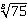Create an Account

Home / Questions / Use the Newton Raphson method and a four function calculator

Use the Newton Raphson method and a four function calculator

. Use the Newton–Raphson method and a four-function calculator (+ − ×÷ operations only) to computewith four significant figure accuracy.

2. Find the smallest positive (real) root of x3 − 3.23x2 − 5.54x + 9.84 = 0 by the method of bisection.

3. The smallest positive, nonzero root of cos h x cos x − 1 = 0lies in the interval (4, 5). Compute this root by Brent’s method.

Jul 27 2020 View more View LessSubscribe To Get Solution